1. Introduction

The package pefectphyloR allows you to reconstruct perfect phylogenies underlying a sample of DNA sequences, at a focal single-nucleotide variant (SNV) in a genomic region of interest. The implementation is based on the partitioning of DNA sequences (or SNV haplotypes) using the classic algorithm of Gusfield (1991), and then further partitioning them using heuristics introduced by Mailund et al. (2006).

This documentation shows how you can reconstruct the partitions underlying a sample of DNA sequences and perform the major functionality in the package.

2. Create hapMat object

To reconstruct a perfect phylogeny, you have to first create an object of class hapMat. createHapMat() allows you to create this new object. To create a hapMat data object, you must specify:

1. hapmat: a matrix of 0’s and 1’s, with rows representing haplotypes and columns representing SNVs.
2. snvNames: a vector of names of SNVs labelling the columns of hapmat.
3. hapNames: a vector of names of haplotypes labelling the rows of hapmat.
4. posns: a numeric vector specifying the physical locations along the chromosome (in base pairs) of SNVs in the columns of hapmat.

To illustrate, we consider a toy example with 4 haplotypes and 4 SNVs.

library(perfectphyloR)
# Haplotype matrix
haplo_mat <- matrix(c(1,1,1,0,
0,0,0,0,
1,1,1,1,
1,0,0,0), byrow = TRUE, ncol = 4)
# SNV names
SNV_names <- c(paste("SNV", 1:4, sep = ""))
# Haplotype names
hap_names <- c("h1", "h2", "h3", "h4")
# SNV positions in base pairs
SNV_posns <- c(1000, 2000, 3000, 4000)
ex_hapMat <- createHapMat(hapmat = haplo_mat,
snvNames = SNV_names,
hapNames = hap_names ,
posns = SNV_posns)
ex_hapMat
## $hapmat ## SNV1 SNV2 SNV3 SNV4 ## h1 1 1 1 0 ## h2 0 0 0 0 ## h3 1 1 1 1 ## h4 1 0 0 0 ## ##$posns
##  1000 2000 3000 4000
##
## attr(,"class")
##  "hapMat"

3. Reconstruct perfect phylogeny at a focal SNV

Once the hapMat object is created, you may reconstruct a perfect phylogeny at a focal SNV with reconstructPP() by specifying the arguments:

1. hapMat: a data structure of class hapMat, created by createHapMat().
2. focalSNV: the column number of the focal SNV at which to reconstruct the perfect phylogeny.
3. minWindow: minimum number of SNVs around the focal SNV in the window of SNVs used to reconstruct the perfect phylogeny.
4. sep: character string separator to separate haplotype names for haplotypes that cannot be distingushed in the window around the focal point. For example, if haplotypes “h1” and “h3” cannot be distinguished and sep = “-”, then they will be grouped together with the label “h1-h3”.

For example, we can reconstruct a perfect phylogeny at the first SNV of the package’s small example dataset, ex_hapMatSmall_data, containing 10 haplotypes of 20 SNVs with:

# load the example hapMat data object

data(ex_hapMatSmall_data)

# Reconstruct first dendrogram
rdend <- reconstructPP(hapMat = ex_hapMatSmall_data,
focalSNV = 1,
minWindow = 1,
sep = "-")

# Structure of rdend

str(rdend)
## List of 6
##  $edge : num [1:6, 1:2] 5 6 6 5 7 7 6 1 2 7 ... ##$ Nnode        : int 3
##  $tip.label : chr [1:4] "1249" "354-1009-2818" "2909" "1904-454-2931-2994-370" ##$ edge.length  : num [1:6] 6 3 3 4 5 5
##  $node.label : NULL ##$ snvWinIndices: int [1:2] 1 5
##  - attr(*, "class")= chr "phylo"
##  - attr(*, "order")= chr "cladewise"

The reconstructed dendrogram showing the partition structure at the first focal SNV is given in figure 1 of section 5. We can extract the positions of the lower and upper limits of the window of SNVs used to reconstruct the partition, rdend as follows:

# Lower and upper limits of the window of SNVs in hapMat used to reconstruct 'rdend'.
ex_hapMatSmall_data$posns[rdend$snvWinIndices]
##  1500 7000

To see the haplotypes in the window of SNVs used to reconstruct rdend, we can use the following command:

ex_hapMatSmall_data$hapmat[, rdend$snvWinIndices:rdend$snvWinIndices] ## SNV3 SNV4 SNV7 SNV9 SNV14 ## 1904 0 0 0 0 0 ## 454 0 0 0 0 0 ## 1249 1 1 1 1 0 ## 2931 0 0 0 0 0 ## 2994 0 0 0 0 0 ## 2909 0 0 0 0 1 ## 354 1 1 1 0 0 ## 1009 1 1 1 0 0 ## 370 0 0 0 0 0 ## 2818 1 1 1 0 0 As can be seen in the above output, there are two groups of haplotypes that have the same ancestral and derived alleles at each SNV position: haplotypes 354, 1009 and 2818, and haplotypes 1904, 454, 2931, 2994 and 370. Therefore, these two groups of haplotypes cannot be distinguished in the reconstructed partition. In the left panel of figure 1, we can verify that the two tips of the partition are comprised of these two groups of haplotypes. 4. Reconstruct perfect phylogenies across a genomic region With the reconsPPregion(), you can reconstruct perfect phylogenies at each possible focal SNV in a hapMat data object. In the following example, we consider the 10 haplotypes of 20 SNVs in the package’s small example dataset, ex_hapMatSmall_data. We reconstruct perfect phylogenies across this subset of SNVs. data(ex_hapMatSmall_data) # Reconstruct partitions across the region. rdends <- reconsPPregion(hapMat = ex_hapMatSmall_data, minWindow = 1) # Structure of the partition for the first SNV. str(rdends[]) ## List of 6 ##$ edge         : num [1:6, 1:2] 5 6 6 5 7 7 6 1 2 7 ...
##  $Nnode : int 3 ##$ tip.label    : chr [1:4] "1249" "354-1009-2818" "2909" "1904-454-2931-2994-370"
##  $edge.length : num [1:6] 6 3 3 4 5 5 ##$ node.label   : NULL
##  $snvWinIndices: int [1:2] 1 5 ## - attr(*, "class")= chr "phylo" ## - attr(*, "order")= chr "cladewise" 5. Plot reconstructed dendrogram You can use plotDend() to plot the dendrogram structure of reconstructed partitions at a focal point. For example, we first reconstruct two perfect phylogenies at the first SNV and tenth SNV of the package’s small example dataset, ex_hapMatSmall_data. We then show the two partition structures of the reconstructed perfect phylogenies with plotDend(). data(ex_hapMatSmall_data) # Reconstruct first dendrogram rdend1 <- reconstructPP(hapMat = ex_hapMatSmall_data, focalSNV = 1, minWindow = 1, sep = "-") # Reconstruct the second dendrogram rdend2 <- reconstructPP(hapMat = ex_hapMatSmall_data, focalSNV = 10, minWindow = 1, sep = "-") par(mfrow=c(1,2), las=1) plotDend(dend = rdend1, direction = "downwards") plotDend(dend = rdend2, direction = "downwards")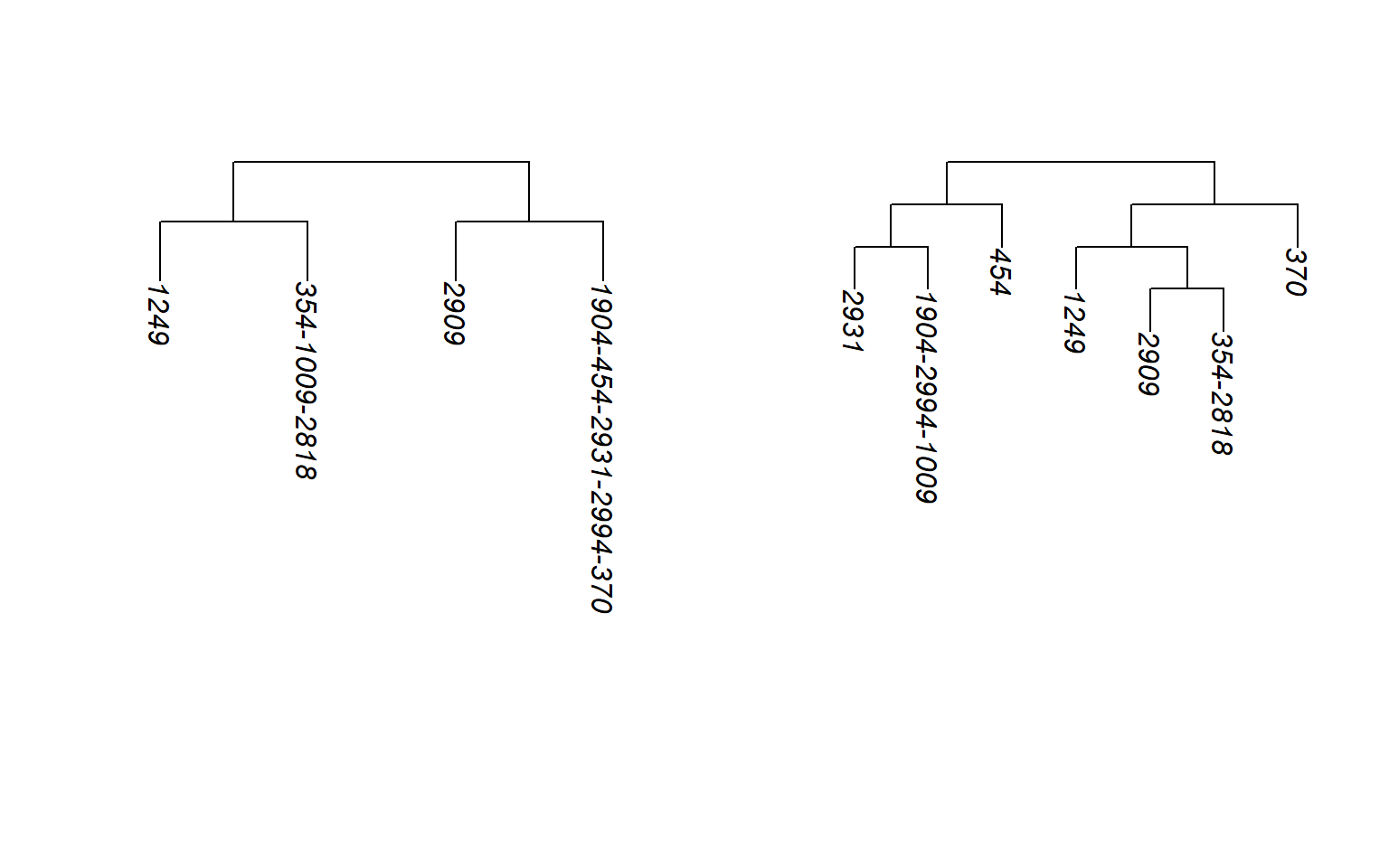Figure 1: Two partition structures reconstructed at the first SNV and the tenth SNV of ex_hapMatSmall_data. In this example, we see that, for the fist SNV, haplpotypes 354,1009 and 2818 cannot be distinguished, nor can 1904,454,2931,2994, and 370. 6. Applications In addition to the reconstruction, this R package allows you to compute five popular measures of association based on the reconstructed partitions, and perform permutation tests of the associations. These association measures include the Rand index (Rand, 1971), dCor statistic (SzÃ©kely et al., 2007), HHG statistic (Heller et al., 2012), Mantel statistic (Mantel, 1967), and RV coefficient (Escoufier, 1970). Application 1: With the function testDendAssoRI(), you can perform the Rand index test of association between a comparator dendrogram, cdend, and a list of reconstructed dendrograms, rdend, across a genomic region of interest. You can use testDendAssoRI() by specifying following arguments: 1. rdend: a multiphylo object of reconstructed dendrograms at each focal SNV. 2. cdend: a phylo object of the comparator dendrogram. 3. hapMat: an object of class hapMatcontaining SNV haplotypes. 4. k : an integer that specifies the number of clusters that the dendrogram should be cut into. The default is k=2. Clusters are defined by starting from the root of the dendrogram, moving towards the tips and cutting across when the appropriate number of clusters is reached. 5. nperm: number of permutations for the test of any association across the genomic region of interest. The default is nperm = 0; i.e., association will not be tested. 6. xlab: an optional character string for the label on the x-axis in the plot that is returned (none by default). 7. ylab: an optional character string for the label on the y-axis in the plot that is returned (none by default). 8. main: an optional character string for title in the plot that is returned (none by default). The function returns the followng components: 1. Stats: a vector of observed Rand indices. 2. OmPval: a permutation-based omnibus P value for the test of any association across the genomic region using the maximum Rand index over the genomic region as the test statistics. 3. mPval: a vector of marginal P values at each SNV position. 4. plt: a plot of the association profile of Rand indices over SNV locations. To illustrate, we use the exmple dataset ex_hapMat_data. We plot the Rand index values summarizing the association between the comparator dendrogram at SNV position 975 kbp and the reconstructed dendrogram at each SNV position across the 2 Mbp genomic region. # Comparator true dendrogram at 975 kbp. data(tdend) # hapMat data object. data(ex_hapMat_data) # Reconstruct dendrograms across the region. allrdends <- reconsPPregion(hapMat = ex_hapMat_data, minWindow = 1) # Rand index profile based on 6 clusters. RI_profile <- testDendAssoRI(rdend = allrdends, cdend = tdend, k = 6, hapMat = ex_hapMat_data, nperm = 1000, xlab = "SNV positions (bp)", ylab = "Rand indices", main = "Association Profile") # Omnibus P value for overall association. RI_profile$OmPval
##  0.000999001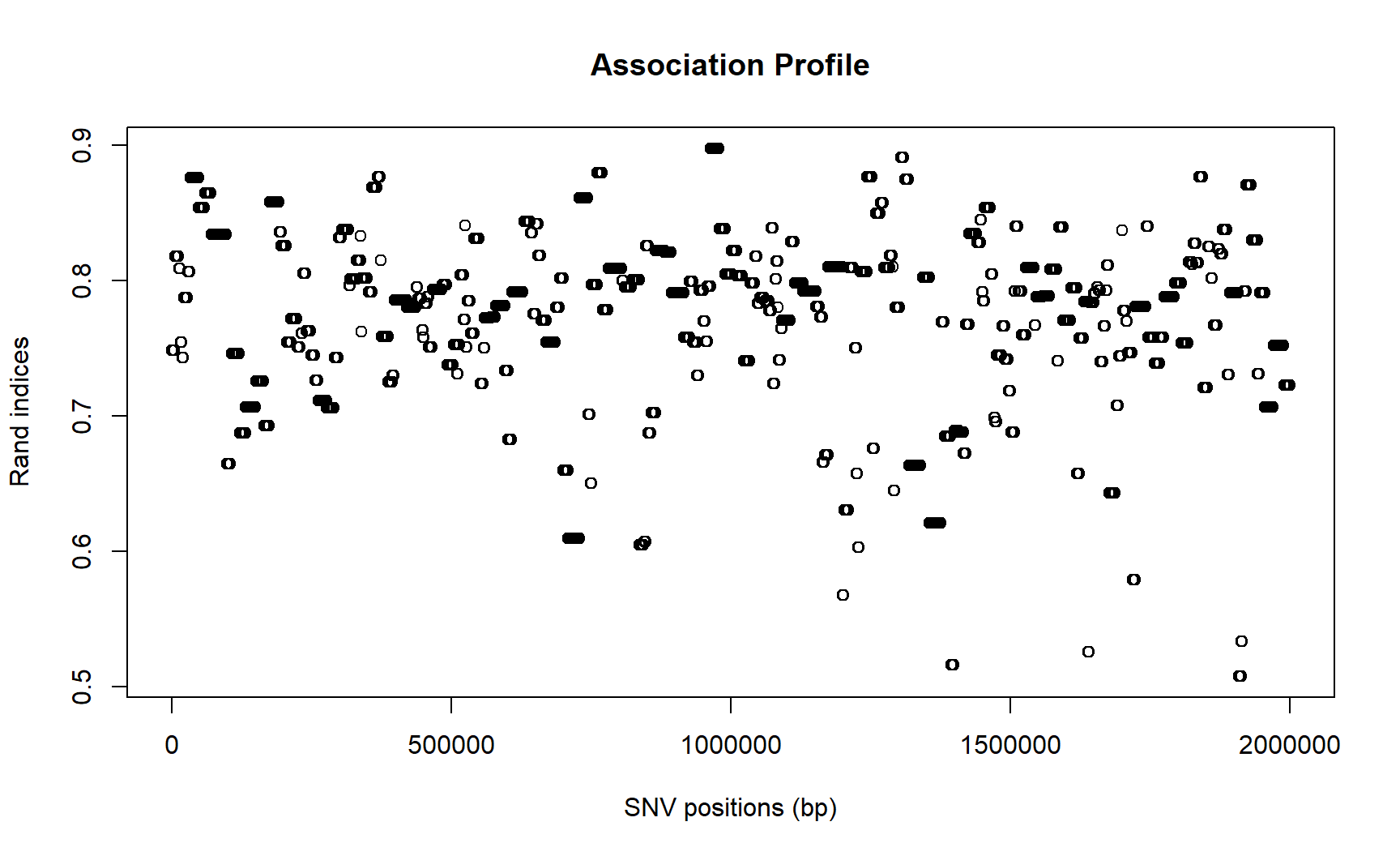Figure 2: Rand index values between the comparator true dendrogram at SNV position 975 kbp and the reconstructed dendrogram at each SNV position across the 2 Mbp genomic region of the example dataset.

As can be seen from the plot, the strongest association is around the SNV position 975 kbp. The Rand index between the true dendrogram at SNV position 975 kbp and the reconstructed dendrogram at the same position is around 0.9. According to the omnibus p value (RI_profile$OmPval), the association across the genomic region is significant. Note that, the function testDendAssoRI() returns NA for the reconstructed partitions that cannot be cut into k clusters. Therefore, you may see some spaces at the positions where the reconstructed partitions are ignored. Application 2: With the function testAssoDist(), you can test the association between a comparator distance matrix, based on any pairwise distance measure, and the reconstructed dendrograms across a genomic region of interest. The association statistics available in the function are the dCor statistic, HHG statistic, Mantel statistic, and RV coefficient. You can perform these association tests by specifying the following arguments: 1. rdend: a multiphylo object of reconstructed dendrograms at each focal SNV. 2. cdmat: a comparator matrix of pairwise distances (e.g. pairwise distances between haplotypes of a comparator dendrogram). 3. method: association methods. Use “dCor” for dCor test, “HHG” for HHG test,“Mantel” for mantel test, and “RV” for RV test. 4. hapMat: an object of class hapMat containing SNV haplotypes. 5. nperm: number of permutations for the test of any association across the genomic region of interest. The default is nperm = 0; i.e., association will not be tested. 6. xlab: an optional character string for the label on the x-axis in the plot that is returned (none by default). 7. ylab: an optional character string for the label on the y-axis in the plot that is returned (none by default). 8. main: an optional character string for title in the plot that is returned (none by default). The function returns the followng components: 1. Stats: a vector of observed statistics computed by the user-provided distance association method. 2. OmPval: a permutation-based omnibus P value for the test of any association across the genomic region using the maximum statistic over the genomic region as the test statistics. 3. mPval: a vector of marginal P values at each SNV position. 4. plt: a plot of association profile of user-provided distance association method over SNV locations. To illustrate, we plot the dCor statistics summarizing the association between a comparator distance matrix,cdmat, and the reconstructed dendrograms across the genomic region of the package’s example dataset ex_hapMat_data. We first compute the pairwise distances between haplotypes based on the comparator true dendrogram at SNV position 975 kbp. These pairwise distances are computed using the function rdistMatrix() which is available in the package. The rdistMatrix() uses the rankings of the nested patitions in the dendrogram to calculate rank-based distance between haplotypes. However, you can use any distance measure of interest for cdmat. We then plot the dCor statistic summarizing the association between the rank-based distance matrix for the reconstructed dendrograms at each SNV position and the comparator distance matrix at SNV position 975 kbp. # Comparator true dendrogram at SNV position 975 kbp. data(tdend) # hapMat data object. data(ex_hapMat_data) # Compute rank-based distances between haplotypes based on # the comparator true dendrogram (tdend) using the function 'rdistMatrix()'. tdendDmat = perfectphyloR::rdistMatrix(tdend) # dCor profile, comparing the association between distance matrix of true dendrogram # (comparator dendrogram) and all reconstructed dendrogram across the genomic region. # dCor_profile <- testAssoDist(cdmat = tdendDmat, rdend = allrdends, method = "dCor", hapMat = ex_hapMat_data, nperm = 1000, xlab = "SNV positions (bp)", ylab = "dCor Statistics", main = "Association Profile") # Omnibus P value for overall association. dCor_profile$OmPval
##  0.000999001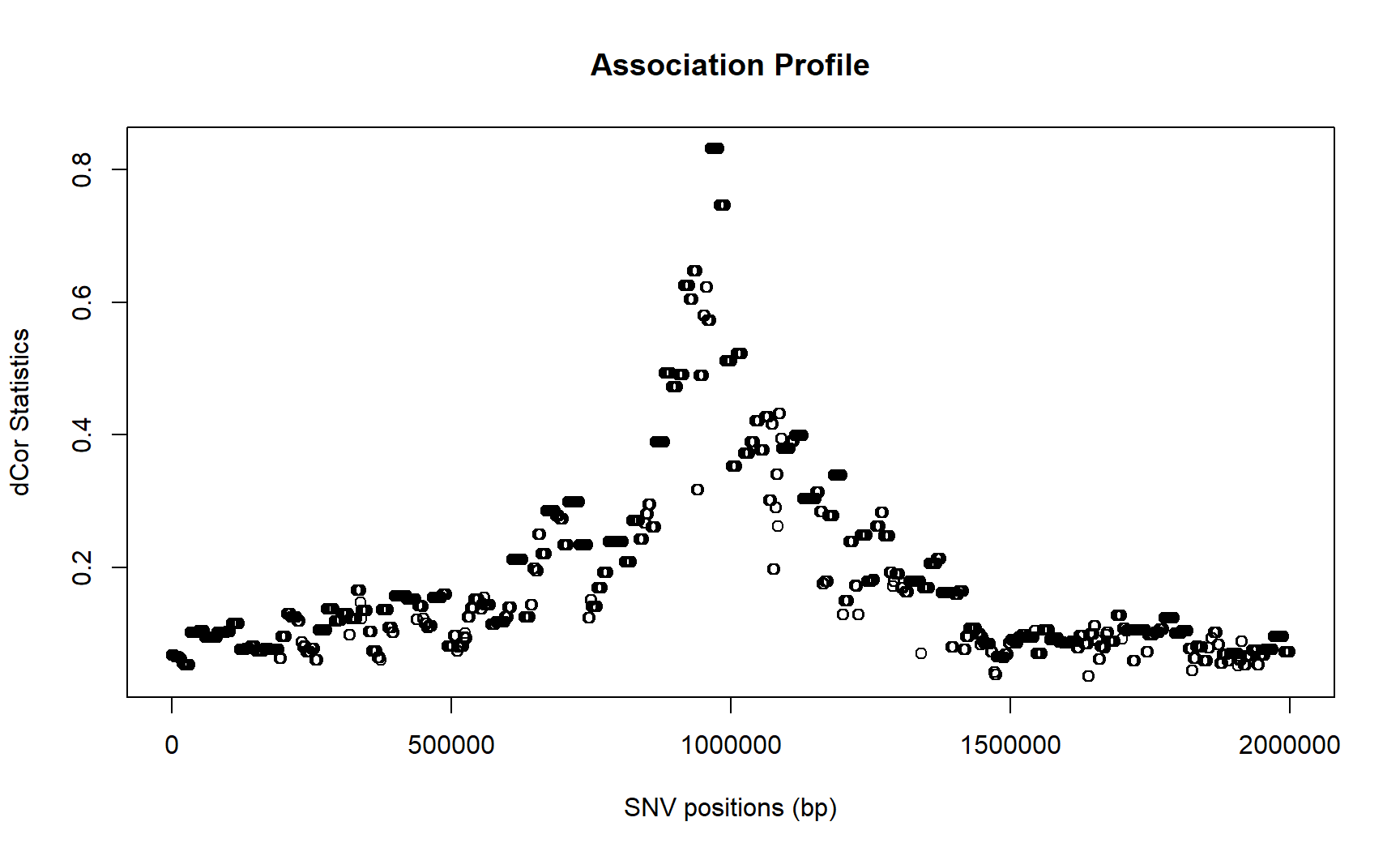Figure 3: dCor statistics between the comparator distance matrix at SNV position 975 kbp, and the reconstructed dendrograms at each SNV position across the 2 Mbp genomic region of the example dataset.

Not suprisingly, the strongest association is found around the SNV position 975 kbp, and it is highly significant.

Application 3:

To illustrate another application of the function testAssoDist(), we perform the RV test of association between a phenotypic distance matrix as the cdmat argument and the reconstructed dendrograms across the genomic region of the example dataset ex_hapMat_data. The disease phenotype data and distances are described in Karunarathna and Graham (2018). These distances are contained in the data object phenoDist. Binary disease status was assigned based on causal SNVs from the middle subregion of 950 - 1050 kbp within a 2 Mbp genomic region of interest.

# Phenotypic distances
data(phenoDist)

# After that compute the RV profile.
RV_profile <- testAssoDist(cdmat = phenoDist, rdend = allrdends, method = "RV",
hapMat = ex_hapMat_data, nperm = 1000,
xlab = "SNV positions (bp)",
ylab = "RV coeffients", main = "Association Profile")

# Omnibus P value for overall association.
RV_profile$OmPval ##  0.4945255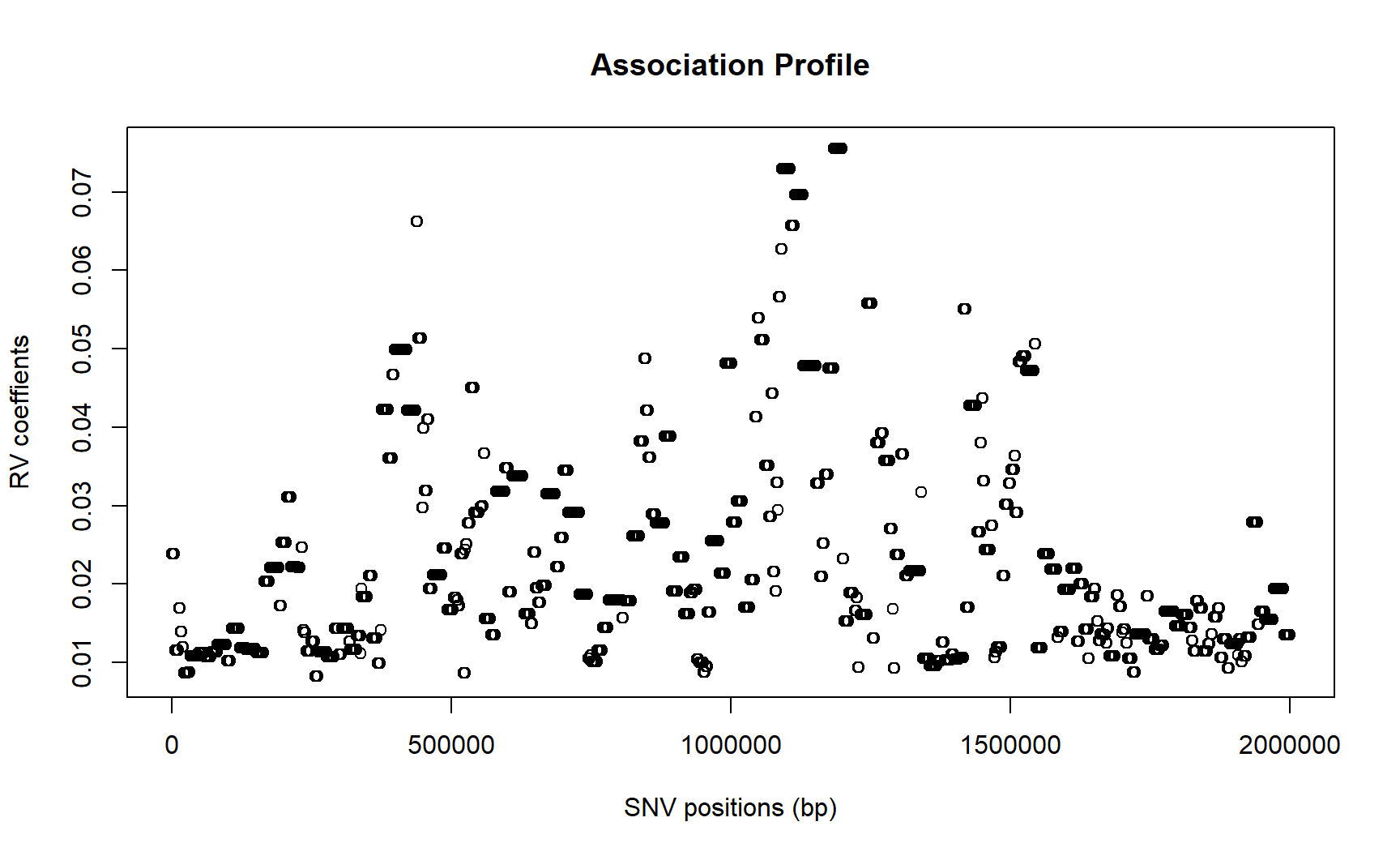Figure 4: RV coefficients between the phenotypic distance matrix and the reconstructed dendrograms across the 2 Mbp genomic region of the example dataset. As can be seen in the plot, the strongest association is close to the middle subregion of 950 - 1050 kbp. But in this example, it is not significant according to the omnibus test for any association across the genomic region of the example dataset ex_hapMat_data(P value $$\approx$$ 0.5). The following example shows how you can indicate the middle subregion of 950 - 1050 kbp where the disease causal SNVs are located (risk region). # plot association profile. plot(ex_hapMat_data$posns, RV_profile\$Stats, main = "Association profile with the risk region"
, xlab = "SNV positions (bp)", ylab = "RV coefficients")
# Indicate the risk region where the causal SNVs are located.
abline(v=950000); abline(v=1050000)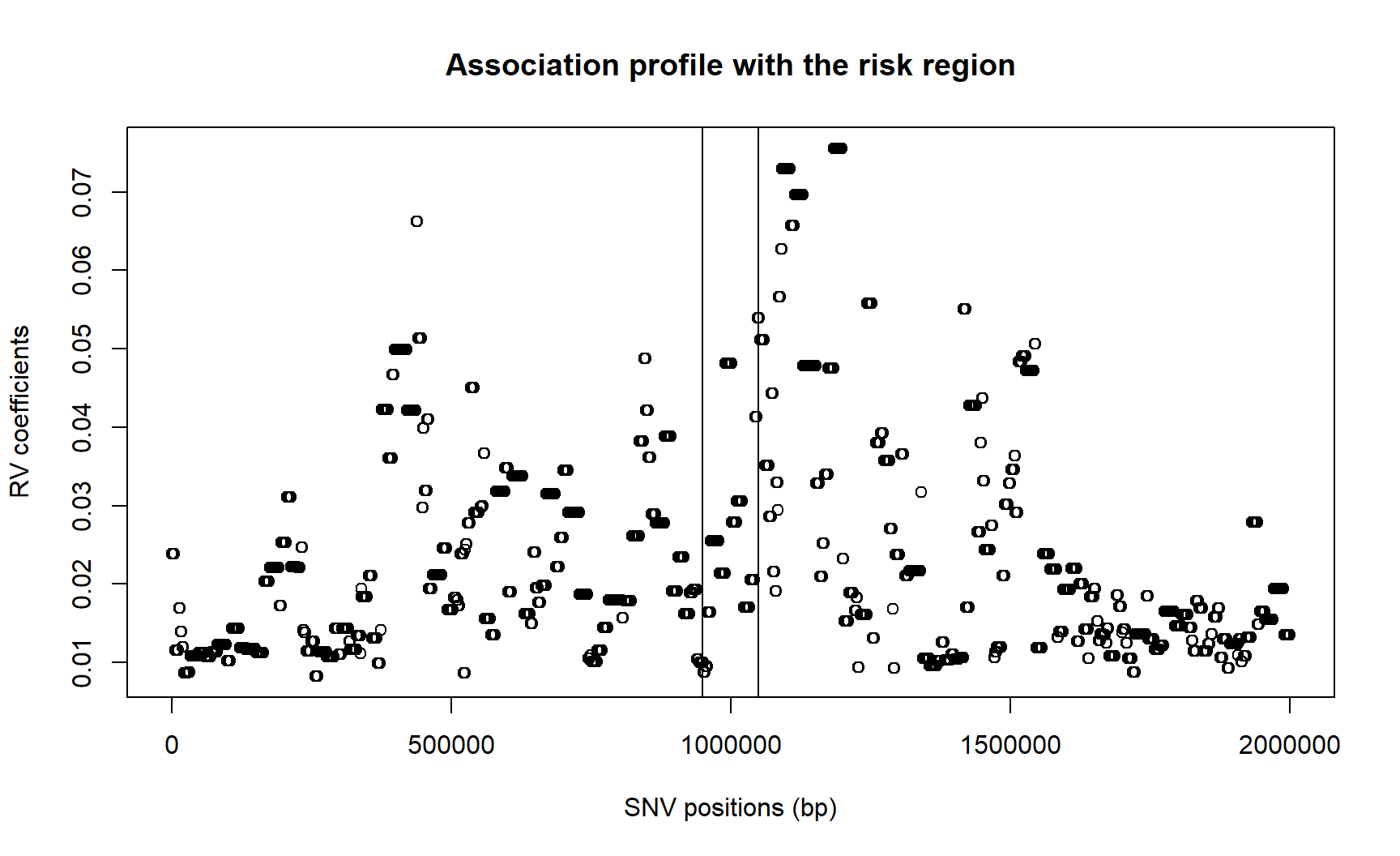Figure 5: RV coefficients between the phenotypic distance matrix and the reconstructed dendrograms across the 2 Mbp genomic region of the example dataset annotated with risk region.

7. Timing

The following table shows the computation times of the package’s major functions in minutes on an Intel E5-2683 v4at 2.1 GHz with 20 GB of RAM. These computation times are for 2747 SNVs with the 200 haplotypes in ex_hapMat_data data set that is included in the package.

Function Time without permutation (minutes) Time with 1000 permutations (minutes)
reconstructPP() Few seconds NA
reconsPPregion() 11.0 NA
testDendAssoRI() 1.0 40.0
testAssoDist() 0.33 236.0